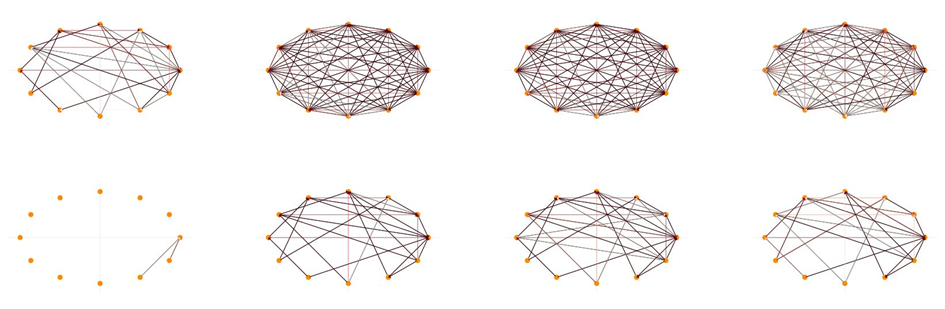# Equivariant Deep Learning for Network Data

Andreas Abildtrup Hansenen: Equivariant Deep Learning for Network Data
In practical applications one will often experience that the representation of data will affect the predictions made by a specific model. When we represent a graph using an adjacency matrix, we implicitly incorporate a specific ordering of the nodes. However, this ordering is somewhat arbitrary, and the choice of another ordering for the construction of an adjacency matrix would be equally good, in the sense that it would be a representation of the same graph. Or if one were to observe points located on a sphere, then rotating every point, given a specific representation, would result in the same distribution of points on this sphere. Another example could be when observing images of a specific object. In this case we don’t care about the placement or the object in the image, or whether the object has been rotated; the object is still the same object. These observations lead us to the realization, that many datasets contain certain symmetries which any model trained using this data must consider.

One can make sure, that models account for the symmetries of the used data by choosing architectures which by construction takes these symmetries into account. As such we want to have an inductive bias build into our model which ensures that certain symmetries are always respected; we want our models to be invariant or equivariant with respect to certain symmetries. It has been shown that several state-of-the-art deep learning architectures includes layers which are invariant/equivariant with respect to some relevant symmetry group

Various mathematical models have proven extremely successful in solving many different graph-based machine learning problems. These problems appear in with many different flavors. Some traditional problems are for instance: Graph-classification, node-classification, graph-regression, or link-prediction. To these can be added a variety of problems which can be seen as a special case of a graph-based problem, e.g., image classification, where the graph in question is just a 2D grid, or various point-set predictions, where the point-set can be seen as a graph with no edges. The appearance of all these gives rise to the question of whether there exists some unifying approach, some unifying framework, which can be used when modelling any problem which can be interpreted as a graph-based machine learning problem. One way of approaching the problem of creating such a framework is to consider graph-to-graph prediction problems where both input and the target to be predicted can be interpreted as a graph. This class of problems has only been sparsely described likely due to its difficulty; in its most general interpretation the input and target graph do not necessary reside in the same domain i.e., there may for instance not be an obvious pairing of nodes between nodes of the input graph and nodes of the output graph.

In this project we will seek to create deep learning models for graph-to-graph predictions which respect the symmetries identified in the data considered.

## PhD project

By: Andreas Abildtrup Hansen

Section: Visual Computing

Principal supervisor: Aasa Feragen

Co-supervisors: Ole Winther, Anna Calissano

Project title: Equivariant Deep Learning for Network Data

Term: 01/01/2022 → 31/12/2024

## ContactAndreas Abildtrup Hansen
PhD student
DTU Compute

## ContactAasa Feragen
Professor
DTU Compute
+45 26 22 04 98

## ContactOle Winther
Professor
DTU Compute
+45 45 25 38 95
https://www.compute.dtu.dk/english/phd/current-phd/phd-visual/andreas-abildtrup-hansen
30 NOVEMBER 2023